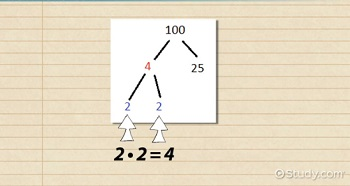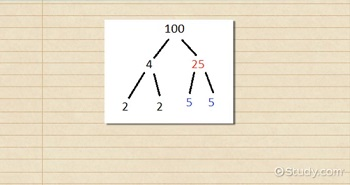# What is a Factor Tree? - Definition & Example

Lesson Transcript
Instructor: Joseph Vigil

Joseph has a master's degree in literature as well as alternative teaching and ESL educator certifications. He has worked with middle school, high school, and college students in writing and language arts.

In this lesson, you'll review factors and prime numbers. You'll learn what a factor tree is and see how it works to reveal any number's prime factorization. Then you can test your new knowledge with a brief quiz.

## Factor Trees and Factors

When we make a family tree, we make a chart of the people that came before us. They're called family trees because they tend to branch out as they progress, as each ancestor gives way to more ancestors. In the same way, a factor tree shows the numbers that come together to create a larger number, and it branches out as it progresses because each resulting factor then gives way to its own factors. In other words, a factor tree is a tool that breaks down any number into its prime factors.

Before we go into detail about factor trees, we need to review factors. Factors are simply numbers you can multiply together to get a certain product. For example, if we're looking at 2 * 3 = 6, then 2 and 3 are factors of 6. But, we could also say that 1 * 6 = 6. So 6 has four factors: 1, 2, 3, and 6. This factor business could get messy with large numbers because, generally speaking, the larger a number is the more factors it will have. That's where the factor tree comes in.

An error occurred trying to load this video.

Try refreshing the page, or contact customer support.

Coming up next: The Box Method for Factoring

### You're on a roll. Keep up the good work!

Replay
Your next lesson will play in 10 seconds
• 0:01 Factor Trees & Factors
• 1:06 Splitting Numbers
• 1:38 Prime Factors
• 3:26 Many Trees, One Prime…
• 4:20 A Large Factor Tree
• 5:28 Lesson Summary
Save Save

Want to watch this again later?

Log in or sign up to add this lesson to a Custom Course.

Timeline
Autoplay
Autoplay
Speed Speed

## Splitting Numbers

For an example of a factor tree, let's start with 100. We can split that number into any of its two factors. In other words, we're going to find two numbers that, when multiplied together, give us a product of 100. Let's start with 4 and 25 because 4 * 25 = 100. And, just like we would in a family tree, we're now going to find the 'ancestors,' or factors, of 4 and 25. We'll start with 4, which we could write as 2 * 2, so we'll put those factors into the tree under 4.## Prime Factors

Now, if we were to break down 2 into its factors, we'd get 2 and 1. Then, we could break down 2 into 2 and 1 again. This could go on infinitely because 1 and 2 are 2's only factors. That makes 2 a prime number, a number whose only factors are 1 and itself. So instead of having an endless chain of twos and ones on the factor tree, we'll stop at 2. In relation to the factor tree, we call the prime numbers prime factors because when all of them are multiplied together they give the original number. We always stop a factor tree's branch at the prime factors to avoid an infinite chain of the same factors.

So we're done with 4's branches. Let's look at 25. We could write that number as 5 * 5, so we'll place those factors on the tree now. Just like 2, 5 is a prime number because its only factors are 1 and 5. Since it's a prime number, we'll end those branches. The factor tree doesn't necessarily produce all of a number's possible factors. For example, 10 is a factor of 100 (10 * 10), but it doesn't show up on the tree.What the tree does give us is a number's prime factorization. A number's prime factorization is simply the list of prime numbers you would multiply together to get a certain product. On a factor tree, all the prime numbers at the ends of branches give us the prime factors. So 100's prime factorization is 2 * 2 * 5 * 5, or 2^2 * 5^2. Every number has one unique prime factorization, just like every person has one unique fingerprint.

To unlock this lesson you must be a Study.com Member.
Create your account

### Register to view this lesson

Are you a student or a teacher?

### Unlock Your Education

#### See for yourself why 30 million people use Study.com

##### Become a Study.com member and start learning now.
Back
What teachers are saying about Study.com
Create an account to start this course today
Used by over 30 million students worldwide